# ISEE Upper Level Math : How to find range

## Example Questions

### Example Question #11 : Range

Find the range of the following data set: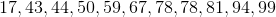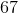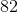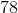Explanation:

Find the range of the following data set:First, let's put our terms in ascending order.Now, find the difference between our first and last terms.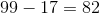So, our range is 82.

### Example Question #12 : Range

Use the following data set to answer the question: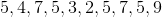Find the range.Explanation:

To find the range of a data set, we will find the smallest and the largest number.  Then, we will find the difference of those two numbers.

So, given the setwe can see the smallest number is 2 and the largest number is 9.  Now, we will find the difference.  To find the difference, we will simply subtract the two numbers. So,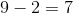Therefore, the range of the data set is 7.

### Example Question #13 : Range

Use the following data set to answer the question: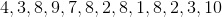Find the range.Explanation:

To find the range of a data set, we will find the smallest and the largest number within the set, then we will find the difference.

So, given the setwe can see the smallest number is 1 and the largest number is 10.  Now, we will find the difference.  To find the difference, we will subtract the two numbers.  So, we get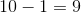Therefore, the range of the data set is 9.

### Example Question #14 : Range

Use the following data set to answer the question: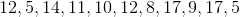Find the range.Explanation:

To find the range of a data set, we will find the smallest and the largest number.  Then, we will find the difference of those two number.

So, given the setwe can see the smallest number is 5 and the largest number is 17.

Now, we will find the difference.  To find the difference, we will subtract the two number.  We get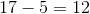Therefore, the range of the data set is 12.

### Example Question #15 : Range

Find the range of the following data set: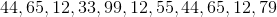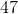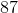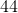Explanation:

Find the range of the following data set:To find the range, first put the numbers in increasing order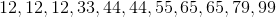Next, find the difference between our first and last terms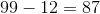Thus, our range is 87 because there is a "distance" of 87 between our biggest and smallest terms.

### Example Question #121 : Data Analysis And Probability

For his last six math tests, Josh scored 92, 80, 88, 94, 97, and 95. What is the range for his test scores?Explanation:

In order to find the range, you will need to first find the smallest and largest number in the set.

For this set of numbers, the smallest number is, and the largest number is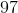.

To find the range, take the difference of these two numbers: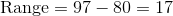### Example Question #17 : Range

Michael received the following scores on his last four French tests: 65, 58, 69, 58. What is the range of his test scores?Explanation:

In order to find the range, you must first identify the smallest and the largest number of the data set.

The smallest number for this set is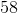, and the largest number is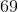.

Take the difference of the two numbers to find the range.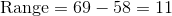### Example Question #18 : Range

Over the course of seven games, Joanna scored the following number of points for her high school's varsity basketball team: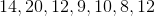.

What is the range of the number of points she scored?Explanation:

In order to find the range, you must first identify the smallest and the largest number of the set.

The smallest number of the set is, while the largest number is.

Take the difference between these two to find the range.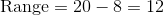### Example Question #19 : Range

Use the following data set to answer the question: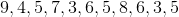Find the range.Explanation:

To find the range of a data set, we will find the smallest number and the largest number.  Then, we will find the difference of those two numbers.

So, given the setwe can see the smallest number is 3 and the largest number is 9.  Now, we will find the difference.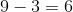Therefore, the range of the data set is 6.

### Example Question #20 : Range

Use the following data set to answer the question: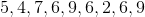Find the range.Explanation:

To find the range of a data set, we will find the smallest number and the largest number. Then, we will find the difference of those two numbers.

So, given the setwe can see the smallest number is 2 and the largest number is 9. Now, we will find the difference.Therefore, the range of the data set is 7.